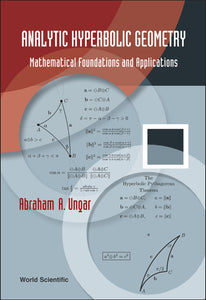# Analytic Hyperbolic Geometry: Mathematical Foundations And Applications

Abraham Albert Ungar

Format: Print Book

ISBN: 9789812564573

• SGD 209.72
Unit price per
Tax included.

This is the first book on analytic hyperbolic geometry, fully analogous to analytic Euclidean geometry. Analytic hyperbolic geometry regulates relativistic mechanics just as analytic Euclidean geometry regulates classical mechanics. The book presents a novel gyrovector space approach to analytic hyperbolic geometry, fully analogous to the well-known vector space approach to Euclidean geometry. A gyrovector is a hyperbolic vector. Gyrovectors are equivalence classes of directed gyrosegments that add according to the gyroparallelogram law just as vectors are equivalence classes of directed segments that add according to the parallelogram law. In the resulting “gyrolanguage” of the book one attaches the prefix “gyro” to a classical term to mean the analogous term in hyperbolic geometry. The prefix stems from Thomas gyration, which is the mathematical abstraction of the relativistic effect known as Thomas precession. Gyrolanguage turns out to be the language one needs to articulate novel analogies that the classical and the modern in this book share.The scope of analytic hyperbolic geometry that the book presents is cross-disciplinary, involving nonassociative algebra, geometry and physics. As such, it is naturally compatible with the special theory of relativity and, particularly, with the nonassociativity of Einstein velocity addition law. Along with analogies with classical results that the book emphasizes, there are remarkable disanalogies as well. Thus, for instance, unlike Euclidean triangles, the sides of a hyperbolic triangle are uniquely determined by its hyperbolic angles. Elegant formulas for calculating the hyperbolic side-lengths of a hyperbolic triangle in terms of its hyperbolic angles are presented in the book.The book begins with the definition of gyrogroups, which is fully analogous to the definition of groups. Gyrogroups, both gyrocommutative and non-gyrocommutative, abound in group theory. Surprisingly, the seemingly structureless Einstein velocity addition of special relativity turns out to be a gyrocommutative gyrogroup operation. Introducing scalar multiplication, some gyrocommutative gyrogroups of gyrovectors become gyrovector spaces. The latter, in turn, form the setting for analytic hyperbolic geometry just as vector spaces form the setting for analytic Euclidean geometry. By hybrid techniques of differential geometry and gyrovector spaces, it is shown that Einstein (Möbius) gyrovector spaces form the setting for Beltrami-Klein (Poincaré) ball models of hyperbolic geometry. Finally, novel applications of Möbius gyrovector spaces in quantum computation, and of Einstein gyrovector spaces in special relativity, are presented.

Format: Hardcover
No of Pages: 484
Imprint: World Scientific
Publication date: 20050907